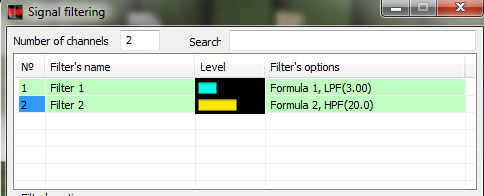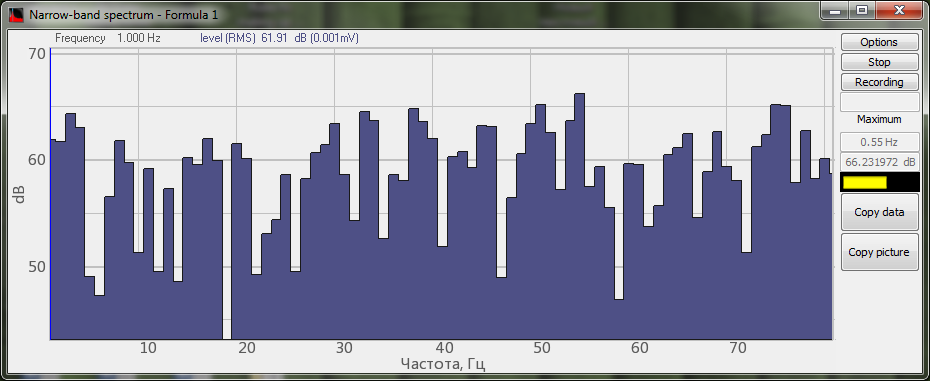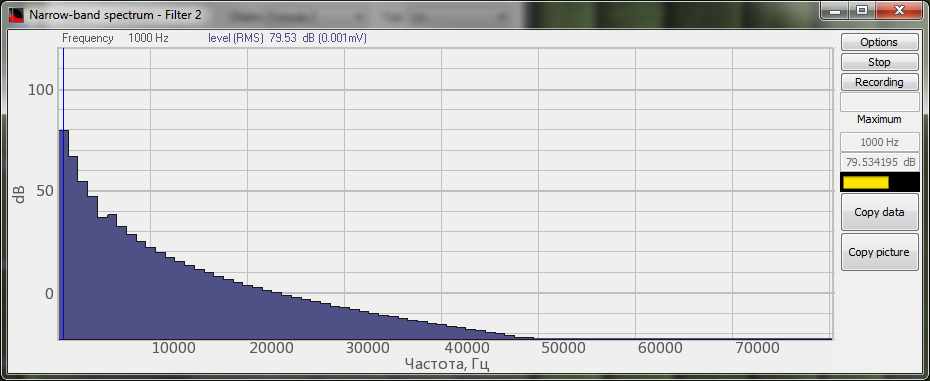# Filters

program Formula

Menu Filters contains functions, allowing to use filters of various types:### Introduction into the use of finite duration impulse response filters

Finite duration impulse response filters have a delay which is determined by the number of coefficients used. The delay is always equal to the amount of the coefficients. The amount of filters is also a criterion of filter operation precision: the ratio of sampling frequency by the channel to the number of filter’s coefficients represents 50% of the frequency band in which the filter meets corresponding requirements. In other words, it is always better to increase the amount of the coefficients used in the digital filter. However, there is a limitation: if there are a lot of coefficients used, the signal filtration consumes a lot of process time. That is why the Formula program has an algorithm used for calculation of the best possible amount of coefficients.

The amount of filter coefficients is calculated in accordance with the following algorithm:

1) it is necessary to calculate the processor speed and the number of the coefficients, so that the load of a single processor core would be 3%. For that purpose, the following formula is used:

where tapslen – is the number of filter coefficients,
CPUSPEED – processor speed, Hz,
ADC_FREQUENCY – sampling frequency for the filtered channel.

2) In the case, if the result obtained exceeds the maximum possible number of the coefficients (2000), there is used an equal number of coefficients.

3) In the case if the result obtained is less than the minimum acceptable (20), then there is used an equal amount of the coefficients.

4) In the case if the data obtained does not allow to obtain an accurate result in terms of frequency, then the following formula is used:

tapslen = 1/(0.5*NORM_FREQ),

where NORM_FREQ – is the normalized frequency value, i.e. the relation of frequency to sampling frequency.

5) In the case if the result obtained implies a high consumption in terms of computation capacity (i.e. there is more than 30% load of a single processor core), then the final result will be calculated in accordance with the following formula:

Example:

Let us calculate the time delay for a channel with the 500kHz sampling frequency of the 2.5 GHz dual-core processor and the 300 Hz LPF used.

The algorithm is as follows:

1) tapslen = 2.5e+9 * 2e-2 / 5e+5 = 100 – in this case the processor load will be 2% divided by the amount of cores, i.e. 1%
2), 3) – are of no relevance
4) tapslen = 1 / (5e-1 * 3e+2 / 5e+5) = 3.3e+3 – the necessary amount of the coefficients to be used for a particular task
5) tapslen = 2.5e+9 * 3e-1/ 5e+5 = 1500 – the maximum possible number of coefficients that can be used in accordance with the specified conditions.

Now, as we have obtained the number of filter coefficients tapslen = 1500, we have to evaluate the time delay as well as compliance of the filter with the accuracy requirements.

Time delay dt = tapslen/ADC_FREQUENCY = 1.5e+2 / 5e+5 = 3e-4 (seconds), or 300 ms.

Filter cut-off frequency: Fcut-off = ADC_FREQUENCY/(0.5e-1 * tapslen) = 5e+5 / ( 0.5e-1 * 1.5e+3) = 6.7e+3 (Hz), i.e. twice as much as is required.

Please, pay attention to the following: if a high sampling frequency is used, there is no need to set the filters with high accuracy, since it may lead to the excessive load of the processor. It is necessary to select optimal frequency range. In the case, if it is necessary to control HF-signal, we recommend you to use hardware heterodyne.

Note:the program Signal filtration uses filters with unlimited pulse characteristics. In the description of Formula filters, there will be used the results of filters operation from Signal filtration program (for comparison purposes).

### Filters description

FIRl(, const) – low-frequency filter with a limited bandwidth (const). The frequency cannot be less than zero or more than 50% of the sampling frequency. This filter type should be used to eliminate excessive high-frequency components, for example, when it is necessary to control the position of a man or a vehicle, i.e. when the controlled signal is within low-frequency range.

FIRh(, const) –  low-frequency filter with a limited frequency (const). The frequency cannot be less than zero or more than 50% of the sampling frequency. This type of filter should be used to eliminate the constant component and low-frequency component of the signal. As an example of the filter implementation, we can consider reproduction of audio information with the elimination of constant and low-frequency components of the signal.

The figures below show an example of noise signal filtration (“formula” function is used) by means of low-frequency and high-frequency filters in Formula and Signal filtration programs.Source signal spectrumSignal spectrum (processed by low-frequency filter of Formula program)Signal spectrum (processed by low-frequency filter from “Signal filtration” program)Signal spectrum (processed by high-frequency filter of Formula program)Signal spectrum (processed by high-frequency filter from “Signal filtration” program)

FIRbp(, const 1, const 2) – bandpass filter passing the frequencies from the first up to the second one. The frequencies values cannot be less than zero or more than 50% of the sampling frequency range. In the case, if these parameters are used, the filter uses the maximum acceptable values instead of them. The filter can be used for highlighting the frequencies that are similar to those of the human voice.

FIRbs(, const 1, const 2) – bandpass filter used to highlight the frequencies between the first and the second frequency. The frequencies values cannot be less than zero or more than 50% of the sampling frequency. In the case, if these parameters are used, the filter uses the maximum acceptable values instead of them. The sequence of frequencies in the equation is of no importance.

The figures below show an example of noise signal filtration (“formula” function is used) by means of band-pass and band-stop filters in Formula and Signal filtration programs.Source signal spectrumSignal spectrum (processed by BPF of Formula program)Signal spectrum (processed by BPF of Signal filtration program)Signal spectrum (processed by band-stop filter of Formula program)Signal spectrum (processed by band-stop filter of Signal filtration program)

FIRInteg() – integrating FIR filter. Its characteristics may prove to be unstable, hence, preliminary use of the high-frequency filter is necessary.

FIRDiff() – differentiating FIR filter

FIRAFCH(, ) – FIR filter with a pre-set form of response.

Heterodyne(, frequency, band) – heterodyne. It is used to highlight a band with Frequency, Hz and Band, Hz parameters and to shift it to the low-frequency environment. The main application area of heterodyne is signal frequency demodulation (the process of shifting a section of the spectrum from high-frequency to low-frequency area – it is used for radio-technical purposes).

SMA(, time)  – it is used for filtering of the exponential moving average, the values of which at each following point are equal to the value of source function for the previous period. The moving average is normally used with the values of time periods for smoothing of the short-term oscillations. The mathematical moving average is one of the resultant types, hence, it may be considered as a low-frequency filter used for signals processing. Since in the course of moving average calculation, the function value is calculated anew every time, and the final array of previous values is taken into consideration, the moving average “moves” or “glides” along the dynamic series (More details).

EMA(, constant) – it is used for exponential average filtration. In the case of the normal exponential moving average, the values of the source function are smoothed; however, it is also possible to smooth the values of the resulting function. The “constant” value is variable within the range from “0” up to “1”. The less is the value, the higher becomes the exponential value (More details).

Median(, Time) – is an effective method of processing of the signals that are exposed to the impact of impulse noise. It is one of the digital filters used for the processing of digital signals and images in order to reduce the noise level. The median filter is a non-linear FIR-filter. The report values of the filter window are arranged in ascending (descending) order. The value located in the middle of the sorted list is displayed as the filter output value. In the case of even number of the counts, the filter value is calculated as an average value of two counts from the middle of the sorted list. The window shifts along the filtered signal and the calculation process is repeated (More details).

Grubbs(, Time, Q) – it is used to expunge the outliers. For this purpose, it is recommended to use the Grubb’s criterion. Statistical Grubb’s method for outliers is based on the assumption that the array of measurements results is subject to normal distribution.

### You may also be interested in…

Delivery terms: CIP, CPT or DAP. Customs clearance and delivery is carried out by the "Cordely" custom company (included in the price of the goods). 1992 - 2023 ZETLAB Company